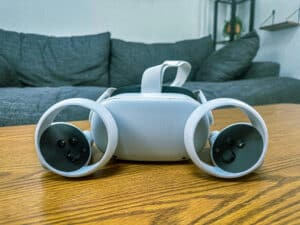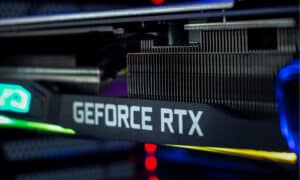### Key Points:

• The 17th century was the beginning of the history of mechanical calculators, the first machine being Pascal’s calculator, which was invented in 1642.
• The mechanical calculator was first invented after three years of research and 50 prototypes.
• In the 18th century, the first mechanical calculator that could perform a multiplication automatically was designed.

## Mechanical Calculators: History

Up to the end of the 17th century, among the most prominent polymaths and encyclopedic scientists of their time—Leonardo da Vinci, Wilhelm Schickard, Blaise Pascal, Gottfried Leibniz—created proposals for the first mechanical calculators, which are recounted in the subsection Pioneers.

The invention of the earliest mechanical calculators, notably Pascal’s calculator, was in the 17th century with the advent of the history of mechanical calculators. Blaise Pascal had constructed a computer he claimed could perform computations previously thought to be only attainable by humans.

### Quick Facts

Created
1642
Creator
Blaise Pascal, Ryōichi Yazu, Abraham Stern, Wilhelm Schikard
Original Use
Addition and multiplication are performed, but not subtraction or division.
Cost
N/A

Some handy tools were invented in the 17th century, aiding arithmetic calculations. This included Napier’s bones, logarithmic tables, and the slide rule, which, because of its simplicity, is still widely used by scientists in multiplying and dividing, ruled over, and hampered the use and development of mechanical calculators until the mid-nineteenth-century release of the arithmometer.

In 1642, Blaise Pascal invented a mechanical calculator with a complex carry mechanism. After three years of work and 50 prototypes, he released his calculator to the world. In the ten years that followed, he created twenty of these machines. Those inventions directly add, subtract, multiply, and divide two numbers and multiply and divide by repetition. This was because the Pascaline dials could only revolve in one direction; unlike Schickard’s machine, zeroing it after each computation required the operator to dial in all 9s and then propagate a carry right through the device (technique of re-zeroing).

This implies that the carry mechanism would have proven itself numerous times in practice. This is a tribute to Pascaline’s quality because none of the machine’s critics in the 17th and 18th centuries mentioned a problem with the carry mechanism. However, it was thoroughly tested on all of the devices by their users, all of the time.

Gottfried Leibniz began research on adding direct multiplication to what he thought was Pascal’s calculator’s operation in 1672. However, it is unlikely that he had ever seen the system in its entirety, and the method could not have worked due to the device’s lack of reversible rotation. As a result, he developed the Stepped Reckoner, a wholly new machine based on his Leibniz wheels, the first two-motion calculator, and the first to include cursors (forming a memory of the initial operand) and had a moveable carriage. In 1694 and 1706, Leibniz constructed two Stepped Reckoners.

Only the machine from 1694 is known to exist; it was revealed in the University of Göttingen at the 19th-century end.

After forty years of development, Leibniz could not construct an utterly functional machine, making Pascal’s calculator the only working mechanical calculator in the seventeenth century. A pinwheel calculator was also described for the first time by Leibniz.

The original mechanical calculator capable of automated multiplication was created in the 18th century, developed and manufactured by Giovanni Poleni in 1709, and constructed of wood. It was the first functional calculating clock. However, like an abacus, they required an operator to select when to cease a repetitive subtraction at each index; hence these machines merely aided in the division; pinwheel calculators and Leibniz wheel calculators were produced with a few failed commercialization attempts.

In 1834, Luigi Torchi developed the first direct multiplication machine. This was also the world’s second key-driven machine, following James White’s.

Thomas de Colmar presented his simpler Arithmomètre, the first machine that could be used regularly in a workplace, in 1851, kicking off the mechanical calculator industry.

The arithmometer was the sole mechanical calculator on the market for 40 years, and it was marketed all over the world.

## Mechanical Calculators: How It Worked

The user-entered data on a set of wheels and an abacus-like layout. The machine could do addition, multiplication, and division, but not subtraction or division. Unfortunately, the structure was mechanically vulnerable, and a “roll-over,” such as from 999 to 1,000, would result in the single tooth cogs failing. Perhaps this is why, some 20 years later, Blaise Pascal was credited with inventing the mechanical calculator.

The most challenging part of creating a mechanical calculator was figuring out how to move a gear proportionally to the amount to be added. Straightforward stylus/slide adders had a simple solution to this. The User merely inserted the stylus into the relevant hole, and the User adjusted the wheel or slide accordingly. However, this was undesirable because the user had no means of knowing whether or not the correct number had been input. In addition, while doing multiplications and divisions, the same number would have to be “dialed in” repeatedly. Designers are compelled to create increasingly complex machines due to these issues.

## Mechanical Calculators: Historical Significance

The purpose of a calculator is to conduct correct computations swiftly. Therefore, it is evident that, to the extent possible, a calculator should relieve the User of the need to perform mental calculations and rely on paper.

A mechanical calculator, also known as a calculating machine, is a mechanical device that automatically performs basic arithmetic operations or (historically) a simulation, such as an analog computer or a slide rule. However, the electronic calculator and the digital computer have rendered most mechanical calculators obsolete, as they were comparable in size to small desktop computers.

Pascal was inspired to construct a calculator due to his father’s job as a tax supervisor in Rouen, which needed arduous arithmetical calculations. He designed the machine to add and subtract two numbers instantly and perform multiplication and division using repetitive addition and subtraction.

## Up Next…

Curta Calculator – Designed by Curt Herzstark, the Curta was an early handheld mechanical calculator.

Microprocessor Explained – Discover the facts and history of microprocessors, how they are made, and how they’ve shaped technological growth over the years.

Eduard Selling – Eduard Selling invented a mechanical calculator called the Nuremberg scissors.

## Mechanical Calculators FAQs (Frequently Asked Questions)

When was Mechanical Calculator invented?

Pascaline, also known as the Arithmetic Machine, was the first calculator or adding device mass-produced and used. Blaise Pascal, a French mathematician, and philosopher developed and built the Pascaline between 1642 and 1644.

What is Mechanical Calculator?

A mechanical calculator, sometimes known as a calculating machine, was a mechanical instrument that performed fundamental arithmetic operations automatically. However, the electronic calculator has rendered most mechanical calculators obsolete, as they were comparable in size to tiny desktop computers.

Who invented the mechanical calculator?

In 1642, Blaise Pascal invented a mechanical calculator with a complex carry mechanism. After three years of work and 50 prototypes, he released his calculator to the world. In the ten years that followed, he created twenty of these machines.

What is an example of a mechanical calculator?

Following are some examples of Mechanical Calculators

Abacus (ca. 2700 BC), Pascal’s Calculator (1652), Stepped Reckoner (1694), Arithmometer (1820), etc.